Reach Us+44-1522-440391
Analyzing Multiple Outcomes: Is it Really Worth the use of Multivariate Linear Regression? | OMICS International
Journal of Biometrics & Biostatistics

Like us on:

All submissions of the EM system will be redirected to Online Manuscript Submission System. Authors are requested to submit articles directly to Online Manuscript Submission System of respective journal.

Analyzing Multiple Outcomes: Is it Really Worth the use of Multivariate Linear Regression?

Rosa Oliveira1* and Armando Teixeira-Pinto2

1Faculty of Medicine, CINTESIS, University of Porto, School of Health Technology Higher, Polytechnic Institute of Porto, Portugal

2School of Public Health, The University of Sydney, Australia

*Corresponding Author:
Rosa Oliveira
Faculty of Medicine, CINTESIS, University of Porto
Central Street Pinheiro-200, 4510-032 Gondomar, Porto, Portugal
Tel: 00351939355143
E-mails: [email protected], [email protected]

Received: September 05, 2015 Accepted: October 26, 2015 Published: October 30, 2015

Citation: Oliveira R, Teixeira-Pinto A (2015) Analyzing Multiple Outcomes: Is it Really Worth the use of Multivariate Linear Regression? J Biom Biostat 6:256. doi:10.4172/2155-6180.1000256

Copyright: © 2015 Oliveira R, et al. This is an open-access article distributed under the terms of the Creative Commons Attribution License, which permits unrestricted use, distribution, and reproduction in any medium, provided the original author and source are credited.

Visit for more related articles at Journal of Biometrics & Biostatistics

Abstract

In health related research it is common to have multiple outcomes of interest in a single study. These outcomes are often analysed separately, ignoring the correlation between them. One would expect that a multivariate approach would be a more efficient alternative to individual analyses of each outcome. Surprisingly, this is not always the case. In this article we discuss different settings of linear models and compare the multivariate and univariate approaches. We show that for linear regression models, the estimates of the regression parameters associated with covariates that are shared across the outcomes are the same for the multivariate and univariate models while for outcome-specific covariates the multivariate model performs better in terms of efficiency.

Keywords

Ordinary least squares; Correlated errors; Generalized least squares; Monte Carlo

Introduction

In biomedical research it is common that the outcome of interest is characterized by multiple variables rather than a single measure per individual. For example, in clinical trials designed to evaluate the safety and effectiveness of drug-eluting coronary stents, in particular, comparing bare-metal stents with drug-eluting stents we may be interested in multiple outcomes such as myocardial infarction, target vessel revascularization, target lesion revascularization, angiographically verified (definite) stent thrombosis, among others.

A common approach when multiple outcomes are present in a study, is to analyze each outcome independently, in a univariate framework, ignoring the most likely correlation between the outcomes and the multivariate structure of the data. At first glance, this approach may seem less efficient than applying multivariate methods, because it ignores the additional information contained on the correlation between the outcomes. Surprisingly, this is not always the case.

In one of his seminal articles, Zellner  introduced the concept of Seemingly Unrelated Regression (SUR) as an extension to the classical multivariate linear regression (MvLR). In the SUR model (2), each outcome of interest is allowed to be associated with it’s own set of covariates. If all the outcomes are modeled using the same covariates, the SUR model reduces to the classic MvLR.

The SUR estimator proposed by Zellner  was shown to be, in the general case, more efficient than the Ordinary Least Squares (OLS) estimator studied the properties. Later, Srivastava  studied SUR model with second-order moments and also found that as the correlation increases, the relative efficiency decreases which agrees with Zellner  study. Breiman  also investigated different procedures in order for SUR to be more efficient than OLS. All authors concluded that if there is no correlation between the outcomes or if the setting is the same as in the classical multivariate linear regression where all the outcomes are modeled using the same covariates, the coefficients estimators in the multivariate setting is the OLS estimator.

The aim of this article is to show the relative efficiency of the SUR estimator when compared to the OLS estimator, in situations where some covariates are shared by all outcomes but some others are outcome specific. In section two we briefly introduce the SUR model and provide an analytical solution for the SUR estimator in the mixed setting where some covariates are shared and some are not, together with a Monte Carlo simulation for several scenarios. In section 3, two real data examples are introduced to illustrate the results and the final section discusses the implication of these results.

Seemingly Unrelated Regression

A SUR model consists of a set of linear regression equations associating different outcomes Y1,..., YK with covariates in which the errors of the different equations may be correlated (model (2)). In fact, the "seemingly unrelated" expression comes from the apparent lack of relationship between the equations, when each outcome has its own set of covariates, but the equations are related by the possibility of correlation between the error terms. Zellner  has shown that separate modeling of the equations results, in the general case, in less efficient estimates.

The SUR model can be compactly written as: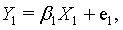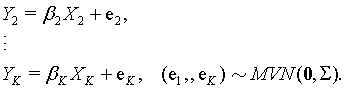(1)

or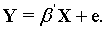(2)

where, Y , is a column vector of the stacked outcome and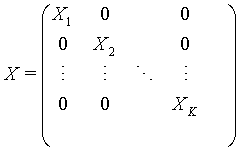Xk representes the design matrix with the set of covariates associated with outcome Yk and β is a column vector of coefficients of explanatory variables and e is a column vector of the error terms.

The usual assumption is that the errors are normally distributed with mean zero and variance-covariance matrix S given by: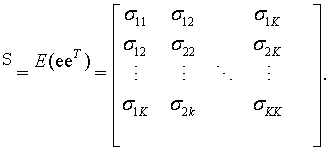In SUR models the several equations may be related by the fact of the errors are correlated across equations; and/or a subset of covariates are the same which allows that each of the K outcomes have a different design matrix with some of the covariates being the same, that has particular relevance.

We first describe a more familiar setting in the biostatistics literature. When the equations share the same set of covariates, i.e., X1 = ... = XK, the SUR model reduces to the classical multivariate linear regression (MvLR). In this setting the error terms associated with each outcome are, again, allowed to be correlated. By ignoring this correlation and fitting K separate regressions this would be equivalent to fitting the SUR model assuming independence of the errors. Therefore, it is obvious that if the errors are not correlated, the SUR estimator is exactly the OLS estimator obtained fitting the K regression separately.

Surprisingly, in the MvLR case (the covariates in each equation are the same) even if the errors are strongly correlated, the SUR estimator always reduces to the OLS. In other words, if the outcomes are modelled using the same covariates, the multivariate model gives the same result (both point estimates and standard errors) as fitting individual regressions for each outcome, despite the level of correlation between the errors. Although this result has been around for many years, it stills raises many eyebrows when is stated, even among experienced biostatisticians. In Appendix A we reproduce the proof of this result.

However, Zellner  has shown that in the case that X1,..., XK are all different there are gains in efficiency by jointly estimating the β in the SUR model over modelling each outcome separately if the errors are correlated. The increase in efficiency is higher if the correlation between the error terms is strong. In particular in his simulations some gains are observed for ρ > 0.3  and explanatory variables in different equations are uncorrelated.

Shared and unshared covariates

We now consider a mixed situation of the two settings described above where the outcomes have some common covariates and other are specific to each outcome. Let’s suppose the multivariate linear model: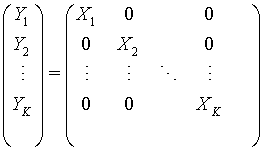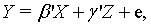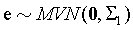where,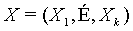represent the vector of each outcome specific covariate for the kth -outcome and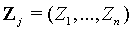the K shared covariates by all outcomes, and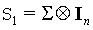with S as previously defined.

One result appears in the particular case when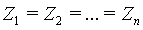. In this case the SUR estimator of β , simplifies to the OLS, this is, if we have the same covariate for all outcomes, the estimator of β that we get from the multivariate model, is exactly the same we get from the univariate regression and, so, the correlation between the error does not play a role in the estimator.

I.e. the correlation between the error terms does not affect the estimation of β and are no efficiency gains when modelling the equations in a multivariate setting compared in applying equation by equation.

Considering what was reported formerly, we want to study the hypothetical gains in the efficiency (SUR compared with OLS) in unshared covariates and in shared covariates coefficients estimates using a multivariate model that takes into account the potential correlation between the error terms when one or more covariates are correlated.

Bearing in mind what was previously reported we expected: to have gains in the efficiency (SUR estimator compared with OLS estimator) in unshared covariates coefficients estimates, and do not have gains in the efficiency of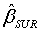when compared with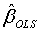in shared covariates coefficients estimates.

Theorem 1 Consider the multivariate linear model:where,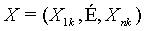represent the vector of each outcome specific covariate for the kth -outcome andthe K shared covariates by all outcomes, and, where S is the variancecovariance matrix.

The best unbiased estimator is given by: =[ X’( ^-1 I_n ) X]^-1 X’( ^-1 I_n ) Y+( Z’ Z)^-1 Z’ Y .

Proof. ={[ X + ( E_K Z) ]’ ( ^-1 I_n )[ X + ( E_K Z) ] }^-1[ X + ( E_K Z) ]’( ^-1 I_n ) Y ={ [ X’( ^-1 I_n ) X ] ^-1+[( E^’_K^-1 E_K )( Z’ Z)] ^-1} [ X + ( E_K Z) ]’( ^-1 I_n ) Y by lemma (1) =[ X’( ^-1 I_n ) X]^-1[ X ( E_K Z) ]’( ^-1 I_n ) Y+[( E^’_K^-1 E_K )( Z’ Z)]^- 1[ X + ( E_K Z) ]’( ^-1 I_n ) Y =[ X’( ^-1 I_n ) X]^-1 X’( ^-1 I_n ) Y+[ X’( ^-1 I_n ) X]^-1( E_K Z)’( ^-1 I_n ) Y+[( E^’_K^-1 E_K )( Z’ Z)]^-1 X’( ^-1 I_n ) Y+[( E^’_K^-1 E_K )( Z’ Z)]^-1( E_K Z)’( ^-1 I_n ) Y for independents X and Z matrices =[ X’( ^-1 I_n ) X]^-1 X’( ^-1 I_n ) Y + [( E^-1_K E_K )^-’( Z’ Z)^-1]( E^’_K Z’)( ^-1 I_n ) Y = [ X’( ^-1 I_n ) X]^-1 X’( ^-1 I_n ) Y_ _SUR+ ( Z’ Z)^-1 Z’ Y_ _OLS (complete proof in Appendix B).

Simulation study

We performed a Monte Carlo simulation study to investigate the efficiency and bias obtained by the univariate and multivariate models, for which the true regression coefficients were known.

For the simulation, we varied the sample size and the correlations between equation errors. 10000 independent samples were generated for the different settings, combining different sample sizes (n=50, n=500, n=1000 and error correlation between the errors (0.0; 0.3; 0.6 and 0.9). Once the 10000 data sets were produced within each correlation range, each set was analysed using both a multivariate linear model, allowing correlation between the error terms, and a univariate linear regression model, ignoring the correlation between the outcomes. The empirical standard errors were obtained by computing the standard deviation of the MLEs for the regression parameters for the set of the simulation. All of the analyses were produced using R 2.11.0 GUI 1.33 Leopard. For each data set, the parameter estimate vectors from the multivariate approach and univariate approach were divided to obtain Relative of the Mean Square Error (RMSDataE).

The model used in each of these data sets was a simple three equation model.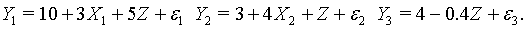Y1 represents the outcomes, Xi the specific covariates for each outcome and Zithe shared covariate by the three outcomes, i =1, 2,3 . Within each outcome, the error term is independently and identically distributed (Appendix C).

X1i was generated from a N(2,3) ; X2i generated from a N(−2,4) and Zi generated from a N(1,1) .

The results, means of the estimates for the regression parameters, of the simulations are summarised in Table 1. Overall, the regression estimates were similar, both for the situation of shared and specific covariates for the outcomes and the empirical standard errors were similar to the average of the standard errors obtained in each simulation.

Coefficients
ρ n=50 n=500 n=5000
β0 β1 β1 β3 β4 β0 β1 β1 β3 β4 β0 β1 β1 β3 β4
0 1.007 1.039 1.001 1.012 1.053 1.001 1 1 1.004 1 1 1.002 1 1 1.002
0.3 1.009 1.039 1.001 1.009 1.03 0.998 0.991 1 0.998 0.989 0.997 0.983 1 0.994 0.973
0.6 0.996 0.996 1 1 0.981 0.993 0.955 1 0.995 0.948 0.991 0.939 1 0.993 0.931
0.9 0.988 0.957 1 0.982 0.928 0.987 0.92 1 0.983 0.882 0.98 0.902 1 0.985 0.884

Table 1: Ratio of the mean square error of the multivariate model (SUR) to the univariate models (OLS) averaged. over the results of 10000 simulated datasets with sample size equal to 50 for each correlation level and for data generated with a common covariate.

In SUR setting, for the particular case of common set of covariates associated with the outcomes, SUR and OLS performed the same and there was no gain in efficiency ofwhen compared with, despite the correlation between the outcomes, reason why de RMSE is omitted in Table 1. However, concerning parameters estimates associated with specific covariates there is a small efficiency gain inwhen compared with. This gain was only about 10% (approx.) and occurred when correlation between the outcomes was high, above 0.6 approximately (Table 1).

We obtained similar parameters estimators for both the approaches, nonetheless on the analysis of the unshared covariates coefficients estimates of the efficiency of SUR estimates compared with equation by equation estimates we obtained efficiency gain when the sample is large, that is, SUR was more efficient than OLS. Curiously, when the sample is small and the correlation low, contradictorily, there is loss in the efficiency ofwhen compared withβ . Concerning the shared covariates, SUR and OLS performed the same and, so, there was not gain in efficiency (reduces to).

Applications

The first example 3.1 illustrates a randomised clinical trial study and shows the similar performance of the approaches described above when the each outcome has one specific covariate and all outcomes share one covariate. The second example, 3.2, illustrates a cohort study looking at cardiovascular risk factors in children. Analogous to the first example each outcome has a specific covariate and both the outcomes share, in this case, two covariates.

Restenosis comparison following coronary stenting using bare-metal stents and drug-eluting stents

Thrombotic events remain the primary cause of death after percutaneous coronary interventions; nonetheless, the growing use of stents has improved the results of percutaneous coronary revascularization . However, bare metal stents cause angiographic restenosis, an undesirable side-effect. Sirolimus-eluting stent implantation, a type of Drug-eluting stents (DESs), were introduced to reduce the incidence of restenosis but there were several reports post drug-eluting stent thrombosis, after more then 360 days.

The data for this example were collected for the SIRolImUS-Eluting Stent in De Novo Native Coronary Lesions (SIRIUS) study. SIRIUS was a randomized, double-bind study conducted in United States with the purpose to assess the safety and efficacy of the sirolimus-eluting stent in the prevention of restenosis in the novo native coronary artery lesions when compared with the uncoated Bx stent.

In brief, the primary endpoint of the study was target vessel failure at 9 months after the procedure. A total of 1101 patients were randomized to either the Cypher sirolimus-eluting stent (n=533) or a bare metal stent (n=525) (43 patients were de-registered).

Procedural success was defined as successful implantation of the study device, a final vessel diameter stenosis <50%. The objective performance criterion was based on three 9 month outcomes: Reference Vessel Diameter (RVD), Post-Stent Diameter Stenosis in Lesion (LPSTDS) and Post-Stent Diameter Stenosis in Stent (SPSTDS) for a more detailed analysis concerning the prediction rule. For each of this outcomes of interest, there are specific covariates, subjects in the study were adjusted for their baselines and a common covariate, all subject in the study shares the group covariate. RVD, SPSTDS and LPSTDS are the specific covariates for RVD9, SPSTDS9 and LPSTDS9 outcomes, respectively. More detailed study design and data collection have been described by Holmes .

By analysing the results, the estimates produced by both OLS and SUR are present in Table 2. Most of the estimates are the same or similar to each other. However, the standard errors for the coefficients of unshared covariates differ by a substantial percentage. For example, LPSTDS residuals are minor correlated with RVD as we saw before, but highly correlated with SPSTDS. Just like we expected there were no significant gains in efficiency in SUR estimate compared with OLS estimate in group coefficient estimate, but there was an efficiency gain in LPSTDS coefficient estimate (approximately 38%). The estimates produced by both OLS and SUR are again approximately the same. Just in SPSTDS coefficient estimate we observe differences.

Intercept Shared Specific
Std Std:E p  value Std Std:E p  value Std Std:E p  value
RVD OLS 0.489 0.046 <0.001 -0.041 0.015 0.007 0.826 0.016 <0.001
SUR 0.512 0.046 <0.001 -0.041 0.015 0.007 0.818 0.016 <0.001
LPSTDS OLS 4.553 0.331 <0.001 1.492 0.108 <0.001 0.217 0.064 <0.001
SUR 4.6 0.214 <0.001 1.477 0.108 <0.001 0.211 0.04 <0.001
SPSTDS OLS 3.109 0.531 <0.001 1.815 0.094 <0.001 0.581 0.078 <0.001
SUR 3.904 0.335 <0.001 1.804 0.094 <0.001 0.465 0.049 <0.001

Table 2: Coefficients estimates and ratio of the mean square error of the multivariate model (SUR) to the univariate models (OLS) in SIRIUS case study results.

More over SPSTDS residuals are minor correlated with RVD as we saw before, but highly correlated with LPSTDS. So, like in previous case, as we expected there were no significant gains in efficiency in SUR estimate compared with OLS Estimate in group coefficient estimate, but there was efficiency gains in SPSTDS coefficient estimate (approximately 38%).

In the coefficients of shared covariates the estimates gains of SUR compared with OLS aren’t exactly zero because errors were assumed as independents but, in the reality, there is some dependence between them (Table 2).

Cardiovascular diseases risk factors in portuguese youngters

The data used in this example were obtained from a large cohort focusing on cardiovascular risk factors in a paediatric population . We focus on one of the longitudinal evaluations of the study and for this example we will use complete and ignore the missing observations. This example does not intend to be a thoroughly analysis of the data or to address a realistic research question. Instead, we build a simple case using known factors that affect blood pressure and contrast the OLS and SUR estimators.

The dataset analysis included 770 children (363 girls and 407 boys). Blood pressure, both systolic and diastolic, demographic and anthropometric measures were available.

We modelled both, systolic (SBP) and diastolic blood pressure (DBP) using age and the z-score of body mass index (BMI). The use of age-standardised BMI is convenient because it is, by construction, independent of the other covariate age. Previous studies [9-13] have found a difference between sexes for the SBP but not for DBP. In this example, the univariate analysis has shown the same relationship, with a significant difference between boys and girls for SBP but not for DBP. Therefore, we used sex as the outcome specific covariate for SBP and we did not include it for the DBP model [14-16]. The final models are then:

SBP = age + sex + BMI DBP = age + BMI

The estimates obtained in this last model using SUR and OLS procedures are presented in Table 3. Most of the coefficient estimates are the same or very close to each other as they are minor correlated and sex becomes significant (Table 3).

Coefficients
Intercept age BMI sex
Std Std:E p  value Std Std:E p  value Std Std:E p  value Std Std:E p  value
SBP OLS 96.862 1.285 <0.001 1.929 0.117 <0.001 3.201 0.285 <0.001 -0.93 0.565 0.0999
SUR 96.933 1.279 <0.001 1.93 0.117 <0.001 3.202 0.285 <0.001 -1.083 0.493 0.0285
DBP OLS 49.1 1.281 <0.001 1.047 0.12 <0.001 1.305 0.29 <0.001
SUR 49.1 1.281 <0.001 1.047 0.12 <0.001 1.305 0.29 <0.001

Table 3: Coefficients estimates and ratio of the mean square error of the multivariate model (SUR) to the univariate models (OLS) in Cardiovascular Diseases Risk Factors in Portuguese Youngsters study results.

The final results presented in Table 3 quantify the associations of the two metabolites expressions that have two shared covariates (age and BMI) and one specific covariate (sex). The results show again that the standard errors of the regression coefficients for specific covariates are about 13% less for the SUR method than for the OLS method. It is worth noticing that the standard errors for the other covariates are the same for the SUR and OLS [17,18].

Conclusion

the present paper two regression methods, multivariate and univariate approach, have been presented to explain a large amount of variance of the prediction. We corroborate that SUR estimator performs better than the equation by equation method of the OLS estimator in estimating a system of regression equations, which are related by their error terms, nonetheless, there were several points regarding these results that deserve special attention. First, it was assumed a normal population, insofar in what way disparities from normality affect the distribution of the coefficient estimators’ remains to be studied. Second, if ρ and if the joint estimation technique is applied, the estimator will have a variance slightly larger then when using separate modeling of the equations in samples of moderate size. Third, it is to be noted that in this paper we investigated a problem for which independents X and Z matrices were considered, that is, X 'Z = 0, which can always happen in real situations. For so, the efficiency of the proposed class has been studied by comparing the two estimators, both with theoretical and practical considerations. With the purpose to validate our study, numerical comparisons were made on one real data set. Improvements upon the regression estimators where achieved and we concluded that the coefficients associated with the outcome-specific covariates there were efficiency gains depending on the correlation between the outcomes. The results have shown that the standard errors of the SUR estimator is lower than the OLS estimator in outcome specific covariates when the errors are correlated between the equations, and are approximately the same in outcome shared covariates despite the correlation between the errors. In other words, there are gains in the efficiency of SUR model over separate equation by equation when contemporaneous correlation between the errors is high. The gains are obtained when correlation for the residuals was more than 0.6 (approximately). Nevertheless, the efficiency gains were minor (even when the correlation was 0.9 the efficiency gain was about 10). Moreover, even if there were efficiency gains in the estimators, we must remember that as the number of comparisons increases, the probability of making, at least, one type I error in the analysis greatly increases, corroborating the idea of performing a single model, SUR model .

Acknowledgements

The authors would like to thank Laura Mauri for SIRIUS data and Jose Mota, Jose Ribeiro and Sandra Guerra (deceased) for Cardiovascular Diseases Risk Factors in Portuguese Youngters who kindly allowed data access selected for the study for helpful comments, which helped to improve the paper. This research was supported in part by a grant from Foundation for Science and Technology (FCT), Rosa Oliveira was supported by Grant PTDC/SAL-ESA/100841/2008.

References

Select your language of interest to view the total content in your interested language

Article Usage

• Total views: 16044
• [From(publication date):
October-2015 - Oct 23, 2019]
• Breakdown by view type
• HTML page views : 12034Can't read the image? click here to refresh